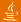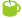### Found in titles:

1.DMelt: jhplot.P1D [100%] (Java API)
2.Jython Example: p1d_operation.py [60%] Operations on P1D data
3.Jython Example: shapes_ellipse1.py [50%] Display an ellipse shape using P1D data points
4.Jython Example: p1d_systematics1.py [50%] P1D data holder with systematical errors
5.Jython Example: p1d_operation1.py [50%] P1D arrays and data manipulations
6.Jython Example: points3.py [50%] P1D 2D array with errors filled as area
7.Jython Example: p2d_contour.py [50%] Using P1D to show data in a contor plot
8.Jython Example: canvas2D_contour2.py [50%] Showing H2D, P1D and 2D function using HPlot2D
9.Jython Example: shapes_ellipse2.py [50%] Display a rotated ellipse using P1D data points
10.Jython Example: p1d_systematics.py [50%] P1D data holder and evaluation of systematics
11.Jython Example: fit_p1d.py [50%] Interactive fit of P1D array and HFit
12.Jython Example: func_minuit.py [50%] Minuit (MnMigrad) minimization of P1D function
13.Jython Example: shapes_ellipse3.py [50%] Display a random ellipse using P1D data points
14.Jython Example: points2.py [40%] Data array P1D and how to show it using HPlot
15.Jython Example: points_oper.py [40%] Using P1D class for data manipulation (with uncertainties)
16.Jython Example: p1d_average.py [40%] Using P1D data holder and calculate weighted average
Searching 62556 articles took 7 ms